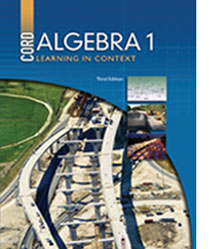Welcome to CORD Online Resources For Learning in Context

Learning in Context is an on-line supplement for our mathematics and science textbooks. Each lesson in CORD’s textbooks is supported by a series of links to various websites. These links add new or more in-depth meaning to the lessons taught in the textbook.

You can navigate each book by using the expanding menu at the left or clicking on the chapters below.

Algebra 1 - 3rd EditionChapters

Chapter 1 :Operations, Expressions and Equations

Chapter 2: Measurement

Chapter 3: Solving Equations

Chapter 4: Linear Equations

Chapter 5: Nonlinear Functions

Chapter 6: Probability

Chapter 7: Statistics

Chapter 8:Systems of Equations

Chapter 9:Inequalities

Chapter 10: Polynomials and Factors